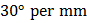# Plane polarized light is passed through a polaroid. On viewing through the polaroid we find that when the polariod is given one complete rotation about the direction of the light, one of the following is observed a) The intensity of light gradually decreases to zero and remains at zero b) The intensity of light gradually increases to a maximum and remains at maximum c) There is no change in intensity d) The intensity of light is twice maximum and twice zero

## Question ID - 150094 :- Plane polarized light is passed through a polaroid. On viewing through the polaroid we find that when the polariod is given one complete rotation about the direction of the light, one of the following is observed a) The intensity of light gradually decreases to zero and remains at zero b) The intensity of light gradually increases to a maximum and remains at maximum c) There is no change in intensity d) The intensity of light is twice maximum and twice zero

3537

The intensity of light is twice maximum and twice zero

Next Question :
 In the visible region of the spectrum the rotation of the plane of polarization is given by. The optical rotation produced by a particular material is found to beatandat. The value of constantwill be a)b)c)d)